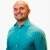# SAT PREP Learn how to determine the final weight given a ratio of weightsThis lesson will look at an example problem involving weight. This lesson will give an explanation of how to determine the final weight given a ratio of weights with a step by step look to better... This lesson will look at an example problem involving weight. This lesson will give an explanation of how to determine the final weight given a ratio of weights with a step by step look to better explain the concept.
More... Collapse
1 Views